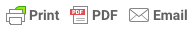# 1.1 Expressions Worksheet Explained

Here is the Expressions Worksheet for Algebra 1 students.  I know, you're thinking - hey, I'm not an idiot, what do you intend to explain?  Please - read on ....  I am labeling this set of Problems 1.1 and it is meant to assess the students understanding of elementary expressions, variables and operation signs.  My intention is that, if you like the worksheet, you will copy and paste it into your own word processor.  You can then make modifications if you like.  We're making an effort to include different types of problems without too much repetition.  The hope is that there will be many many worksheets, at least one for every conceivable  topic included in Algebra 1.

The buttons below allow you to print, make a PDF, or email the following worksheet.  If you float your mouse over the worksheet you will see that you have the option to click and remove details (like this explanation).  I got this application from a place called Print Friendly.  They are doing the advertising when you choose to print, PDF, or e-mail.  Seems fair as they are letting me use the code for free.

## More about the Expressions Worksheet

I've kept the worksheets down to about 24 questions, and I will be trying to use different formats for the questions.  Repetition is considered good.  But, I have found that a lot of students won't do a lot of problems.  If they can answer these worksheets by themselves - they know the material.  If you want repetition - there's always the problems in the text book.  You have the option of erasing material you don't want included in your worksheet (like this information).  However, what the heck - I've included a clean version ready for printing at 101 Expressions.

So, you realize, if I include an answer sheet, then your students will have an answer sheet.  I see no way around this.  But, I am certainly open to suggestions.

Please note that all fields followed by an asterisk must be filled in.
 Yes - but not too often.www.MyHSMath.com

Worksheet 1.1 - Expressions Worksheet

Vocabulary

Terms - Parts of an expression.  A term is numbers and variables that are separated by plus, negative, equal or non-equality signs.

Expression - Numbers, operators, and variables grouped together to form part of an equation.  An expression does not contain a variable.

-------------------------------------------------------------------

Part 1  -  Name the operation used in the expression.

1)  5 - c                                   2)  6 + d                                    3)  2/e

4)  5(f)                                    5) 4 ÷ g                                     6)  2*h

7)  4k                                     8) m - 2                                     9) n/5

Part 2  -  Evaluate the expression for the given value of the variable (replace the variable with the number it is representing.

10)  7 + x when x = 3                              11)  16/a when a = 2

12)  0.5b when b = 3                              13)  5 - y when y = 2

14) Bob drives to work every day at an average speed of 30 mph. How far does he drive in two hours?

Part 3 -  Let x = 2, y = 3, and z = 4.  Substitute these variables into the following expressions and evaluate the expression.

15)  z - x                                       16)  xy                                 17)  z ÷ x

18)  x + y                                      19) x*z                                 20) z/y

Part 4 - Matching - Draw a line from each word to the appropriate expression.

21)                   Product                                                          A)   a - b

22)                   Sum                                                                B)  b ÷ a

23)                   Quotient                                                        C)  ab

24)                  Difference                                                    D)  a + b

www.MyHSMath.com